# Marlon

Marlon drew a scale drawing of a summer camp. In real life, the sand volleyball court is 8 meters wide. It is 4 centimeters wide in the drawing. What is the drawing's scale factor?

Simplify your answer and write it as a ratio, using a colon.

Result

M =  0.005
r =  1:200

#### Solution:

$a=8 \ m \rightarrow cm=8 \cdot \ 100 \ cm=800 \ cm \ \\ b=4 \ \text{cm} \ \\ \ \\ M=b/a=4/800=\dfrac{ 1 }{ 200 }=0.005$
$r=M=0.005=\dfrac{ 1 }{ 200 }=1:200$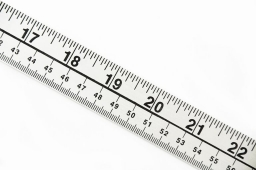Our examples were largely sent or created by pupils and students themselves. Therefore, we would be pleased if you could send us any errors you found, spelling mistakes, or rephasing the example. Thank you!

Leave us a comment of this math problem and its solution (i.e. if it is still somewhat unclear...):Be the first to comment!Tips to related online calculators
Need help calculate sum, simplify or multiply fractions? Try our fraction calculator.
Check out our ratio calculator.
Do you want to convert length units?

## Next similar math problems:

1. What time is it?What time is it, when there is 4 times less time left until midnight than the time that has elapsed since noon? What time is it, when one-fifth of the hours that have passed since midnight is equal to one-third of the hours that are missing by 12 o'clock?
2. The dough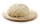The dough contains water, flour, and sugar. Water and flour in a ratio of 2: 3, flour, and sugar in a ratio of 2: 1. Determine the ratio of all three components of the dough.
3. Age of familyThe age of father and son is in the ratio 10: 3. The age of the father and daughter is in ratio 5: 2. How old are a father and a son if the daughter is 20?
4. Brass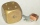Brass is an alloy of copper and zinc in a ratio of 3: 2. How many grams does a component that required 270 g of copper weigh?
5. Interior anglesCalculate the interior angles of a triangle that are in the ratio 2: 3: 4.
6. Job applicantsJob applicants: three-fourths of applicants had experience for a position. The number that did not have prior experience was 36. How many people applied for the job?
7. LibraryNew books were purchased for the library. Five-eighths were professional books, one-fifth were encyclopedias, and 231 books were dictionaries. How many professional books were there?
8. The angles ratioThe angles in the ABC triangle are in the ratio 1: 2: 3. find the sizes of the angles and determine what kind of a triangle it is.
9. Lookout towerCalculate the height of a lookout tower forming a shadow of 36 m if at the same time a column 2.5 m high has a shadow of 1.5 m.
10. ChestnutsThree divisions of nature protectors participated in the collection of chestnut trees.1. the division harvested 1250 kg, the 2nd division by a fifth more than the 1st division and the 3rd division by a sixth more than the second division. How many tons of
11. Garden exchangeThe garden has the shape of a rectangular trapezoid, the bases of which have dimensions of 60 m and 30 m and a vertical arm of 40 m. The owner exchanged this garden for a parallelogram, the area of which is 7/9 of the area of a trapezoidal garden. What is
12. A bucketA bucket has 4 liters of water in it when it is 2/5 full. How much can it hold?
13. Simplest form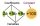Find the simplest form of the following expression: 3 to the 2nd power - 1/4 to the 2nd power
14. Ducats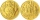The king divided the ducats to his three sons in a ratio of 2: 5: 4. How many ducats the king divided them if the youngest received 260 ducats, which was the least of all sons.
15. A library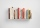A library has 12,500 fiction books and 19,000 non fiction books. Currently 2/5 of the fiction books are checked out. Currently 2/5 of the non fiction books are checked out. Of the books checked out, only 1/10 are due back this week. How many books are due
16. LunchLunch is given to seniors from 12:15 to 12:40 during the Coronavirus pandemic. What angle will the minute hand of clock describe during this time?
17. Profitable companyThree businessman decide to open up their own company. They agree to distribute the yearly profits made in the same ratio as their initial investments. They invest R 50 000, R 75 000 and R25 000, respectively. The profit made by the company in the first y